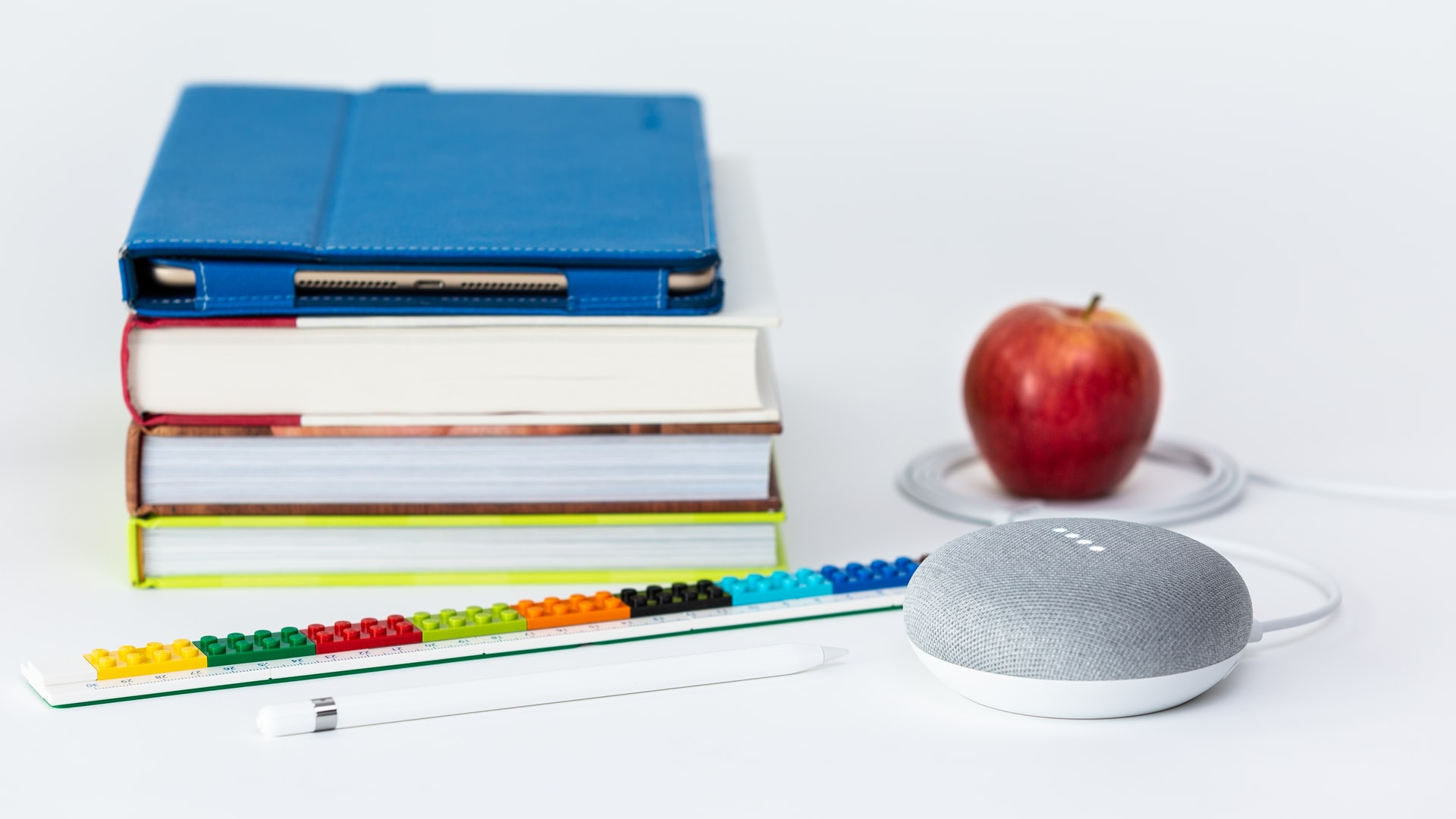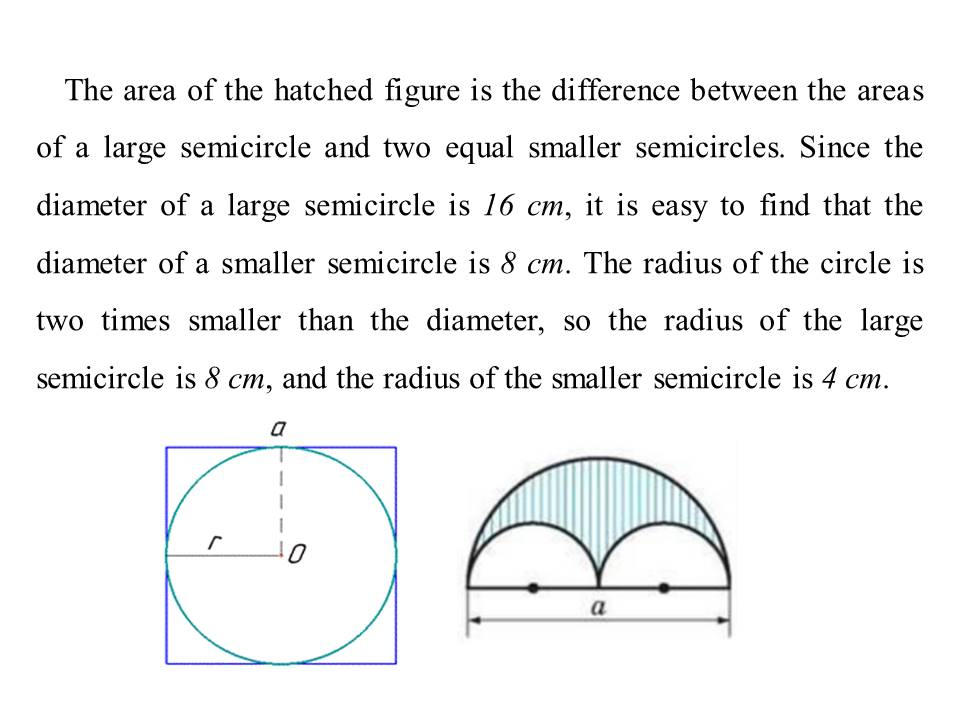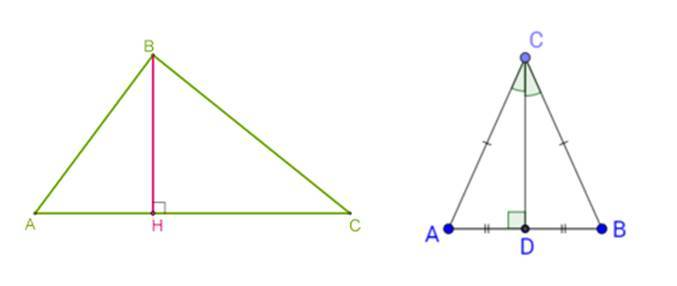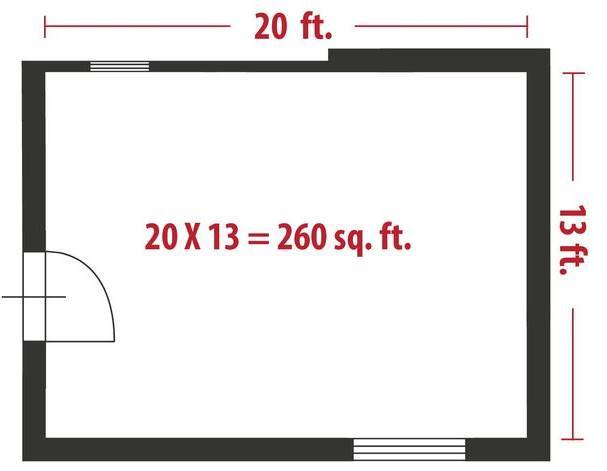Glory to Ukraine!Support Ukraine »

# How to Calculate Square Footage?

16.09.2022School education

This is not only the main mathematical topic, but also one of the most practical and popular things in everyday life. For example, you need to know the floor area in your room to determine how much parquet it will need. The theme of the square is relevant in construction, engineering, architecture, sculpture, drawing and even solving everyday issues.

Next, you will learn how to calculate the areas of the main figures, how to find out the total footage of your home and much more.

## How to Calculate the Area of a Square?By the way, you can find a good private teacher for yourself or your child with the help of UpskillsTutor. It is one of the best tutoring sites in the USA.

It is also worth considering the rectangle (and most of the objects on the terrain are usually rectangular in shape). It has a width (a) and a length (b). The area value is determined quite simply: a*b

How big is a square foot in inches? You may wonder about this and similar transitions. So, 1 square foot equals 144 square inches, and m²=ft²/10.764.

## Square Footage of a Circle

It is pretty easy to calculate if we know the radius. We are also going to need the number π. So, the area is calculated by multiplying: 3,14*r2

Let us look at a more complex problem. Calculate the area of the hatched figure if a=16cmThe area of the large semicircle is π*r2/2=82 π /2=32π.

Two smaller semicircles form a circle, so the square measurement is π*42=16π. Thus, we have a difference of 32π–16π. So the area of the hatched figure is 16π.

## Square Footage of a Triangle

To calculate the area of a triangle, its height (denote it h) and the length of one of the sides (a) are used. And sq ft of triangle is ½*a*hAs for the right triangle, we need its sides, which form a right angle. Let's call them a and b. So, the area will be equal to ½*a*b.

And now consider the equilateral triangle (each side is a). In this case, the area value is calculated as (a2*√3)/4.

## How to Figure Square Footage of a House?

To measure the area, it is necessary to measure the length (a) and width (b) of the walls in each room. This is done with a tape measure, and it is best to use a construction tape because it has a fairly large length and perfectly holds a flat line. All measurements are taken accurately along the walls.

When the room measurements are finished and everything is recorded, you can proceed with the calculations. Formula for sq feet: а*b. Now add all the results obtained by the area of each room – it will be the total living area of your house or apartment.And what if the rooms are, for example, irregularly shaped? A math tutor will help you figure out how to solve practical problems. 99% of private lessons are practice, and the one that will be needed in professional and everyday life.

### Read more: The Most Common English Slang Words & Phrases

Rate the article

Rate: 5 of 5

Based on reviews from 1 users

Need a tutor?

Choose the best teachers on the UpskillsTutor service!